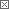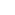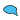Challenge Questions Blog### Challenge Questions

So do you have a Challenge Question that could stump the community? Then submit the question with the "correct" answer and we'll post it. If it's really good, we may even roll it up to Specs & Techs. You'll be famous!

Answers to Challenge Questions appear by the last Tuesday of the month.

 Previous in Blog: River Sailing: Newsletter Challenge (09/13/05) Next in Blog: Bathroom Mirror: Newsletter Challenge (09/27/05)# Water Can: Newsletter Challenge (09/20/05)

Posted September 20, 2005 7:00 AM

The question as it appears in the 09/19 edition of Specs & Techs from GlobalSpec:

The kids are trying an experiment. Taking a can filled with water, they are going to put 3 holes in the side of the can, spaced at equal intervals up one side at heights H/4, H/2, and 3H/4. Keeping water flowing into the can to maintain a constant water level H , they are arguing about which stream of water will travel the farthest horizontal distance. Your son says the stream from the top hole will go the farthest because the water drops the farthest and therefore must spend the most time in the air. Your daughter says, "No, silly. It's the water from the bottom hole that will go the farthest, because it gets the most pressure from all the water in the can." You let them argue for a while, listening to the rationale for each of their views, and then weigh in with the answer. What is it?

Click here to view previous Challenge Questions. Check back next Tuesday afternoon for the answer to this puzzler and a new Challenge Question.

Interested in this topic? By joining CR4 you can "subscribe" to#1

###This is tough

09/20/2005 3:23 PM

I assume no air resistance, so the water will won't lose any of it's velocity in the x-direction (parallel to the ground)till it hits the ground. So it sounds like a ballistics problem with different intial velocities and different heights. Since the water from all three will be accelerated in the y-direction (perpendictular to the ground) by g. The time each will spend in the air before hitting the ground will be sqr(2h/g). So that would be the time it had to travel in the x-direction for a given x. I don't know how to calculate the initial velocity of due to pressure so I'm stuck.

Anyone have any ideas?

Participant

Join Date: Sep 2005
Posts: 2#2

###Re:This is tough

09/20/2005 11:54 PM

The velocity is proportional to the square root of the pressure difference inside and outside the vessel. The pressure at the height h is Rg(H-h), where R - is the density of water. So, the distance is proportional to the Sqr(Rh(H-h)). To find the h that delivers maximum distance, we will take a derivative of Sqr(Rh(H-h)) over h and equate it to zero, which gives us the value of h we are looking for. The derivative is (H-2h)/2sqr(h(H-h)), which gives us the maximum of h=H/2. Cheers.

Guru

Join Date: Jul 2005
Location: Stoke-on-Trent, UK
Posts: 4329#3

###Re:This is tough

09/21/2005 4:22 AM

Don't agree with this. Rg(H-h) is OK, but in distance proportional, g has changed to h. There is no max horizontal distance if allowed to fall far enough (obviously ignoring things like air resistance and evaporation)

__________________
Give masochists a fair crack of the whip
Participant

Join Date: Sep 2005
Posts: 2#5

###Re:This is tough

09/21/2005 8:46 AM

This is just plain algebra. To determine the distance you have to multiply horizontal speed by the time. Your sqr is multiplied by my sqr like this sqr(2h/g) * sqr(Rg(H-h)) = sqr((2h/g)(Rg(H-h))) = sqr(2hR(H-h)). Since 2 is always constant I ignored it. I did not say "equal" I said "proportional". And there is a maximum distance as a function of h. Because at the h=H the distance is zero (no pressure, no speed), as well as at the h=0 the distance again is zero (no time to create horizontal distance). So somewhere in between there is the value of h to deliver a maximum.

Guru

Join Date: Jul 2005
Location: Stoke-on-Trent, UK
Posts: 4329#6

###Re:This is tough

09/21/2005 10:29 AM

I see where you're coming from now - this calc gives horizontal distance to hit a horizontal plane thru tank bottom (at h = 0), and as you say there is a max when h = H/2. But I don't think this is the question. The way I read it it's asking which hole gives max horizontal distance after any vertical fall. Any comment from setter?

__________________
Give masochists a fair crack of the whip
Guru

Join Date: Jul 2005
Location: Stoke-on-Trent, UK
Posts: 4329#4

###Re:This is tough

09/21/2005 4:39 AM

This is pretty much it. You don't need to calculate exactly the (horizontal) velocity from each hole to get the answer, only that it's higher the lower the hole (greater pressure). So the bottom hole goes furthest. But velocity = C*sqrt(2*g*h) where C = discharge coeff, ~ 0.62, and h = height below water surface. Note - bottom hole furthest only applies when drops have fallen a minimum distance. For a horizontal plane say just below bottom hole, the bottom jet hits it nearest wall.

__________________
Give masochists a fair crack of the whip
Power-User

Join Date: Jun 2005
Posts: 104#7

###Question Designed with Answer

09/21/2005 10:58 AM

There would be no point in figuring out which stream ends up at a further horizontal distance at some great distance below the can, because it's obviously going to be the lower one which has more pressure behind it. In school, I learned that questions like these were constructed with a given answer in mind and not to simply answer a question of life. This question really only has context if you figure that the distance traveled is measured at height = 0. In which case, H/2, and someone already got that right. Cheers.

Guru

Join Date: Jul 2005
Location: Stoke-on-Trent, UK
Posts: 4329#10

###Re:Question Designed with Answer

09/22/2005 12:25 PM

It might be fairly obvious to the right sort of engineer, but even he would need to think a short while and remind himself that the horizontal velocity stays constant while the vertical increases. Plenty of other people would have no idea! And how do we know what the context is if the question doesn't mention it? Or what the questioner's motives were?

__________________
Give masochists a fair crack of the whip
Power-User

Join Date: Jun 2005
Posts: 104#13

###Re:Question Designed with Answer

09/22/2005 1:48 PM

True, the question should be stated more clearly and with what assumptions. I have seen this question before, however, and from going to school to the practical world, that questions such as these are designed to be solved by math and don't require so much fluid dynamics or details. Questions in books have to have answers in the back or there's no way to check yourself and if there is not answer then it usually isn't in a book. That's the point I'm making that it shouldn't be bewildering to attack, just try to look at it as simply as you can. No punn intended.

Guru

Join Date: Jul 2005
Location: Stoke-on-Trent, UK
Posts: 4329#14

###Re:Question Designed with Answer

09/23/2005 5:02 AM

OK, and I must admit the h = 0 case is more interesting, a touch of calculus being needed!

__________________
Give masochists a fair crack of the whip
Active Contributor

Join Date: Jul 2005
Posts: 17#8

###Brute force method

09/21/2005 11:05 AM

Assume no terminal velocities, no air resistance, no friction, etc (you know, typical physics class): The distance the water stream will travel is based on the velocity times time (v*t). The time is determined by the acceleration due to gravity and the vertical distance involved (t=SQRT(2h/g). By substitution, we now have d=v*SQRT(2h/g). The velocity of the water is proportional to the amount of water above it (v~SQRT(h)). By substituting again (and changing to a proporation we have: d~SQRT(h)*SQRT(2h/g). Since it's a proportion, we can eliminate the constants to get: d~SQRT(h)*SQRT(h) or simply d~h! There are three holes. The top hole has .25H water above it, the middle has .5H and the bottom has .75H. Ergo, the top hole will travel some distance "d", the middle hole will travel twice that distance and the bottom hole will travel thrice the distance of the top hole.#9

###Re:Brute force method

09/22/2005 9:14 AM

This seems to be the closest in my mind to correct. Can you just explain why the velocity is proportional to the amount of water above it? What equation is that from? I'm not very good at fluid dynamics and I'd be interested to learn.

Active Contributor

Join Date: Jul 2005
Posts: 17#12

###Re:Brute force method

09/22/2005 1:38 PM

Torricelli's Law states that water in an open (cylindrical) tank will flow out through a small hole in the bottom with the velocity it would acquire in falling freely from the water level to the hole. There are a number of constants (density of the fluid, size of the exit hole, surface area of the tank since it's cylindrical) that can be eliminated and the result can be expressed as v = SQRT(2gh) where v is the exit velocity, g is the acceleration due to gravity and h is the height above the hole of the fluid in the tank. Starting there, the rest is plugging in terms.

Guru

Join Date: Jul 2005
Location: Stoke-on-Trent, UK
Posts: 4329#11

###Re:Brute force method

09/22/2005 12:52 PM

I think you're mixing up 2 different h's. For calculating velocity from hole, h is distance of hole below water surface. But for time of fall after leaving the hole, h is distance of droplet below hole. So you can't multiply them and clear the sqrt. I calculate that due to the sqrt(h) in the velocity calc, for distances below the tank base >> hole separation, the horizontal distance is ~ sqrt(hole submergence), not linear with hole submergence.

__________________
Give masochists a fair crack of the whip
Active Contributor

Join Date: Jul 2005
Posts: 17#15

###Re:Brute force method

09/23/2005 12:01 PM

Had to think about that for awhile. You're right - my bad. So, we cannot combine the terms and the relationship doesn't hold. The new result for initial distance is: d ~ SQRT(h)*SQRT(y) where d is the initial(!) horizontal distance, h is the height of the water above the hole and y is the distance of the hole above the ground. Agreed? In that case, I believe that the bottom hole shooting the farthest will hold for all containers whose total height is less than four times the distance the bottom of the container is above the ground. Interestingly, as the overall height of the container increases above this "magic value", the middle hole will begin to shoot further. But, no matter how big h gets relative to 4*y, the top hole never gets the longest distance. Haven't thought through the reasons for that, yet. Gotta be something wrong in there - what am I missing, now?

Guru

Join Date: Jul 2005
Location: Stoke-on-Trent, UK
Posts: 4329#16

###Re:Brute force method

09/26/2005 7:03 AM

Agreed. Also the next bit. On question of top hole furthest - it helps to think of a fixed tank height with varying height Ho of tank base above ground, rather than other way round. Then you can consider negative values of Ho i.e. ground level above tank base. When Ho = -H/4, top and middle holes go same distance (analogous to situation you mentioned when Ho = H/4 and middle and bottom holes coincide). For ground level higher than this, say Ho = -H/3, top hole goes further. I graphed it on MathCad to help to visualise it but it could be done on XL or similar.

__________________
Give masochists a fair crack of the whip
Friend of CR4

Join Date: Dec 2004
Posts: 1995#17

###And the Answer is...

09/27/2005 10:09 AM

As written in the 9/27 issue of Specs & Techs from GlobalSpec:

First, you congratulate them on some good, decent thinking. It is true that the water in the upper stream spends more time in the air. It just doesn't go very fast so it won't get far. It is also true that the bottom stream has the most pressure and therefore comes out of the hole with the greatest speed. It just doesn't spend much time in the air before hitting the ground so it doesn't go far either. This points out why they both need to take a physics course - you can let first principles tell you what's going on! It can be shown (see the links below) that the flow speed out of one of the holes a distance h below the surface is the same as the speed an object would have if it were dropped from the same height h, or v = sqrt(2gh) where g is the gravitational constant. (That in itself is pretty astonishing and is called Torricelli's Law. It is derived using energy considerations.) Given how high the hole is above the ground, you can also determine how long the fluid will remain in the air. (It's the same time it would take a rock to fall the same distance and is equal to sqrt(2(H-h)/g) in our example here, where H is the water surface height and h is the distance from that surface to the hole.) Multiplying the two together - horizontal speed times time in the air - gives horizontal distance traveled and is an equation that says something remarkable: The maximum distance achieved is that from the flow from the middle hole! In fact, the holes at H/4 and 3H/4 end up at the same point and short of the max!

The kids' intuitions were good as far as they went, but the right answer was in between for the same intuitive reasons: the flow from the middle hole has enough velocity, and is high enough above the ground to be airborne awhile, so as to beat either extreme. (Think of the real extremes to see this even better.) The equations tell the story. For a detailed look, check out the link below:

https://demoroom.physics.ncsu.edu/html/ demos/196.html

__________________
Off to take on other challenges. Good luck everybody! See you around the Interwebs.